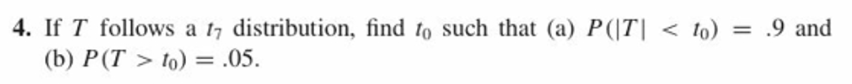# 4. If T follows a 17 distribution, find to such that (a) P(T| to) = .05.

Question
3 viewshelp_outlineImage Transcriptionclose4. If T follows a 17 distribution, find to such that (a) P(T| <。) = .9 and 0 (b) P(T > to) = .05. fullscreen
check_circle

star
star
star
star
star
1 Rating
Step 1

It is given that T follows a t distribution with  degree of freedom. Here we need to find t0 such that

P(|T|<t0) = 0.9

Step 2

here we need to find t0 such that P(|T|<t0) = 0.9. This means that there exists t0 sucht that from -t0  to t0  the distribution has an area of 0.9 as shown in below diagram.

As we know the T distirbution is symmetric we know the area to the right of t0 is half of reamaining area which half of 0.1. So it is 0.05 as shown in the diagram.

Step 3

P(-t0 < T < t0  ) = 0.9

&nbs...

### Want to see the full answer?

See Solution

#### Want to see this answer and more?

Solutions are written by subject experts who are available 24/7. Questions are typically answered within 1 hour.*

See Solution
*Response times may vary by subject and question.
Tagged in

### Statistics